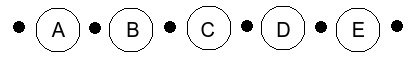# Find the Number of Stopping Stations using C++

There are n number of intermediate train stations between point X and Y. Count the number of different ways trains can be arranged to stop at s stations such that no two stations are next to each other. So in this article, we will explain every possible approach to find out the number of stopping stations. Looking at the problem, we can find that we need to find combinations by which trains can be stopped at s number of stations.

## Approaches to Solve the Problem

Let's take an example that there are eight intermediate stations and we need to find the ways trains can be stopped at three intermediate stations.

n = 8, s = 3

We have (n - s), i.e., five stations left on which train cannot stop,We have five stations A, B, C, D, E, on which trains cannot stop. Now we have six dotted places to arrange three stopping stations such that no two stations are consecutive. Hence, the number of ways are −

6c3= [fact(6) - fact(3)] / fact(3) = 6 * 5 * 4 / 3 * 2 * 1 = 20

There are 20 ways to arrange three stopping stations from points X and Y. So here is the example −

Input : n = 15 s = 4
Output : 495
Input : n = 8 s = 3
Output : 20

## Example

#include<bits/stdc++.h>
using namespace std;
int main(){
int n = 8, s = 3;
int flag1 = 1, flag2 = 1, temp = s, ans;
// selecting 's' positions out of 'n-s+1'
int x = n - s + 1;
while (x != (n - 2 * s + 1)) {
flag1 = flag1 * x;
x--;
}
while (temp != 1) {
flag2 = flag2 * temp;
temp--;
}
ans = flag1 / flag2;
if ((n - s + 1) >= s)
cout << "Number of ways : " << ans;
else
cout << "not possible to find";
return 0;
}

## Output

Number of ways : 20

## Explanation of the Above Code

To understand this C++ code, we can divide the solution into steps.

• Taking the number of stations in n and stopping stations in s as input.

• Initializing flag1 and flag 2 variables with 1 and storing the value of s in a temp variable.

• Calculating flag1 which is numerator [fact(n) - fact(r)].

• Calculating flag2 which is denominator [fact(r)]

• Printing result.

## Conclusion

In this article, we solve a problem to find the number of ways trains can be stopped on intermediate stations such that no two stations are consecutive. We also learned the C++ program for this problem and the complete approach by which we solved this problem. We can write the same program in other languages such as C, java, python, and other languages.